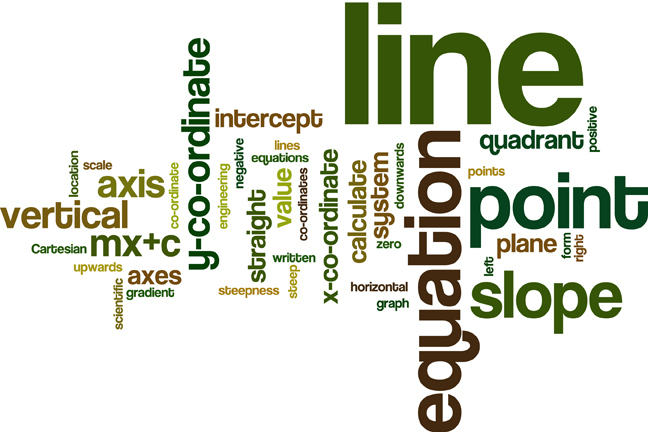2.27

## Loughborough University# Summary of Week 2

We now summarise all that we have learned:

We started by explaining how the location of a point in a plane can be specified precisely by establishing an $x$ - $y$ co-ordinate system, that is, a Cartesian co-ordinate system.

We learned that by drawing a pair of axes at right angles to each other, the plane is divided into four sections called quadrants. Using the scales on the axes and knowledge of the particular quadrant in which a point lies, it became possible to specify a point’s location precisely using co-ordinates $(x,y)$.

Once we were able to plot points, we were then able to join them to produce straight lines.

We learned that the equation of a straight line lying in a plane can be written in the form $y = mx + c$ and that equations like this one relate the $y$ co-ordinate to the $x$ co-ordinate of a point on the line.

Equivalently, any equation of the form $y = mx + c$ has a graph which is a straight line.

The following observations are particularly important:

• The value of $m$ in the equation $y = mx + c$ is called the slope or the gradient of the line.

• If $m$ is positive the line slopes upwards, looking from the left to the right.

• If $m$ is negative, the lines slopes downwards.

• If $m$ is zero, the line is horizontal.

• The numerical value of $m$ is a measure of the steepness of the line.

• The larger the numerical value of $m$ the steeper the line will be.

• The value of $c$ in the equation is called the vertical intercept. It is the $y$ co-ordinate of the point where the line crosses the vertical axis.

Next week we will build upon what we have learned. We will learn how to calculate the slope and intercept of a line passing through two given points, how to calculate the equation of a line with given slope and passing through a given point, and we will introduce letters other than $x$ and $y$ to represent our lines. This is common practice in scientific and engineering applications as we shall see.